# NCERT Exemplar Class 12 Physics Solutions for Chapter 15 Communication Systems

NCERT Class 12 Physics Exemplar Solutions for Chapter 15 Communication Systems provided here are crafted by experts after extensive research on every topic to provide apt and authentic information to the students. If you go through previous years’ question papers, you will get a clear picture of questions in the exemplar book asked in the examination. To score good marks, students need to solve exemplar questions regularly.

The NCERT Class 12 Physics Exemplar for Chapter 15 PDF presents different types of questions like MCQs, fill in the blanks, match the following, true or false, short answer questions, along with numerical problems, important formulas, exercises and assignments. This will help you to understand the concepts clearly and memorise the topics for a long period of time.

## Download the PDF of the NCERT Exemplar for Class 12 Physics Chapter 15 Communication Systems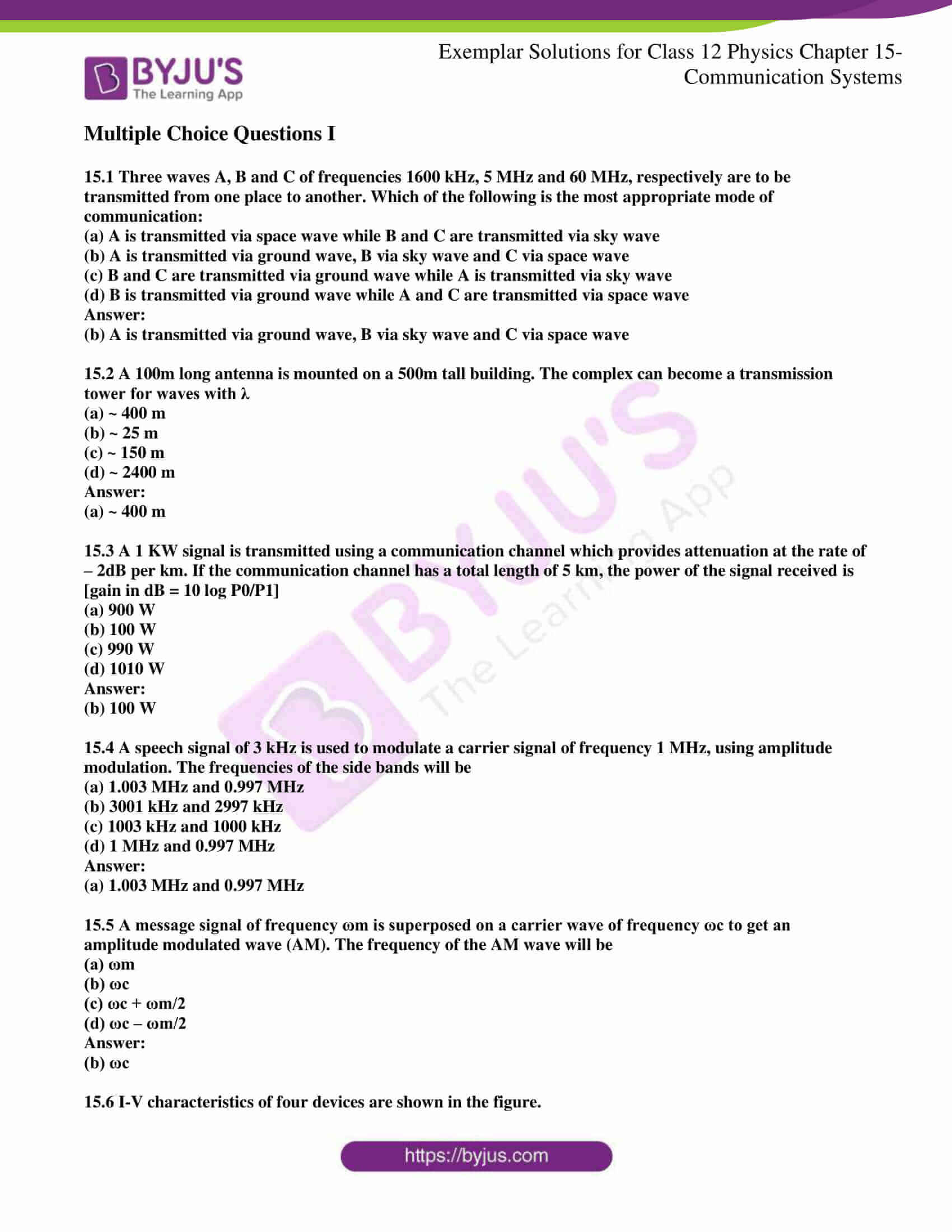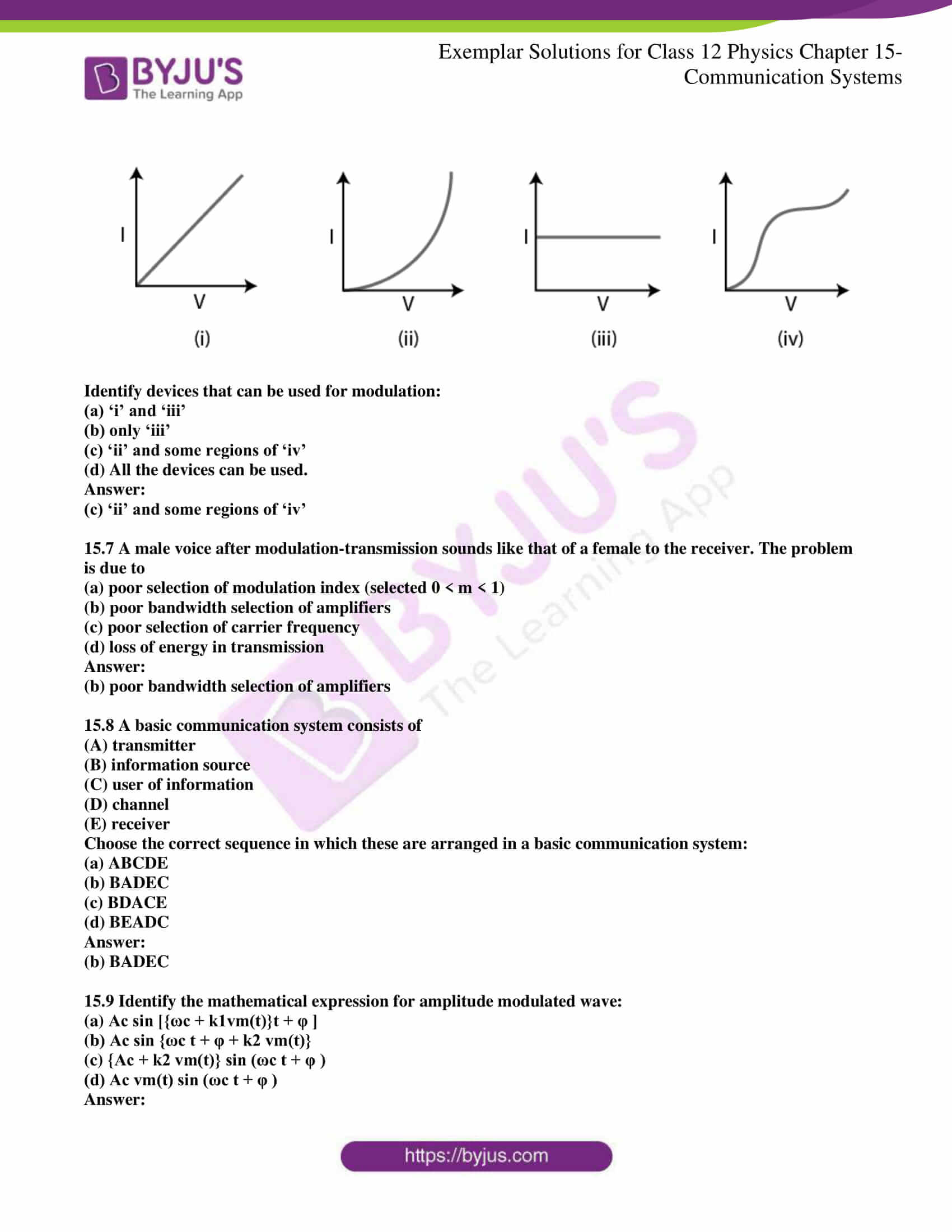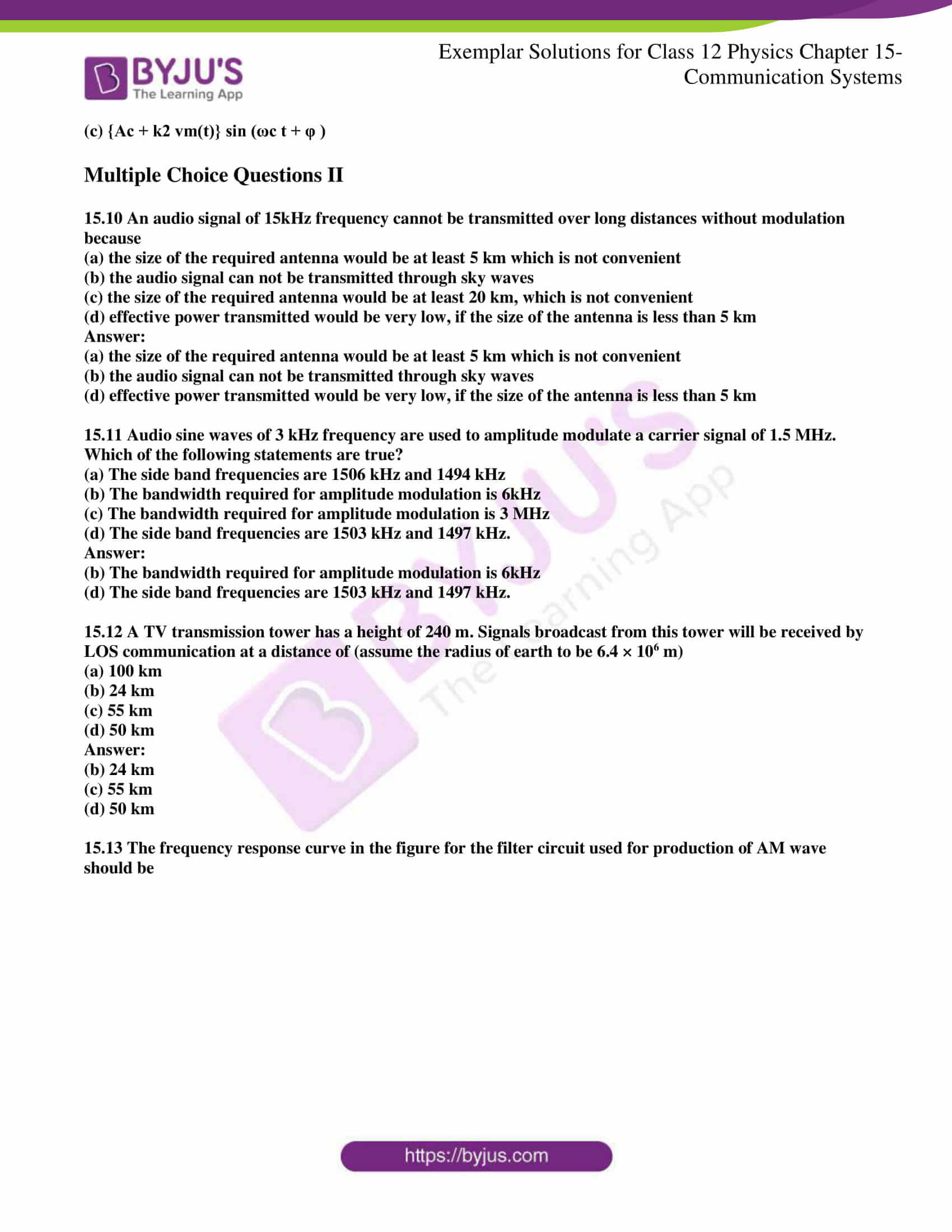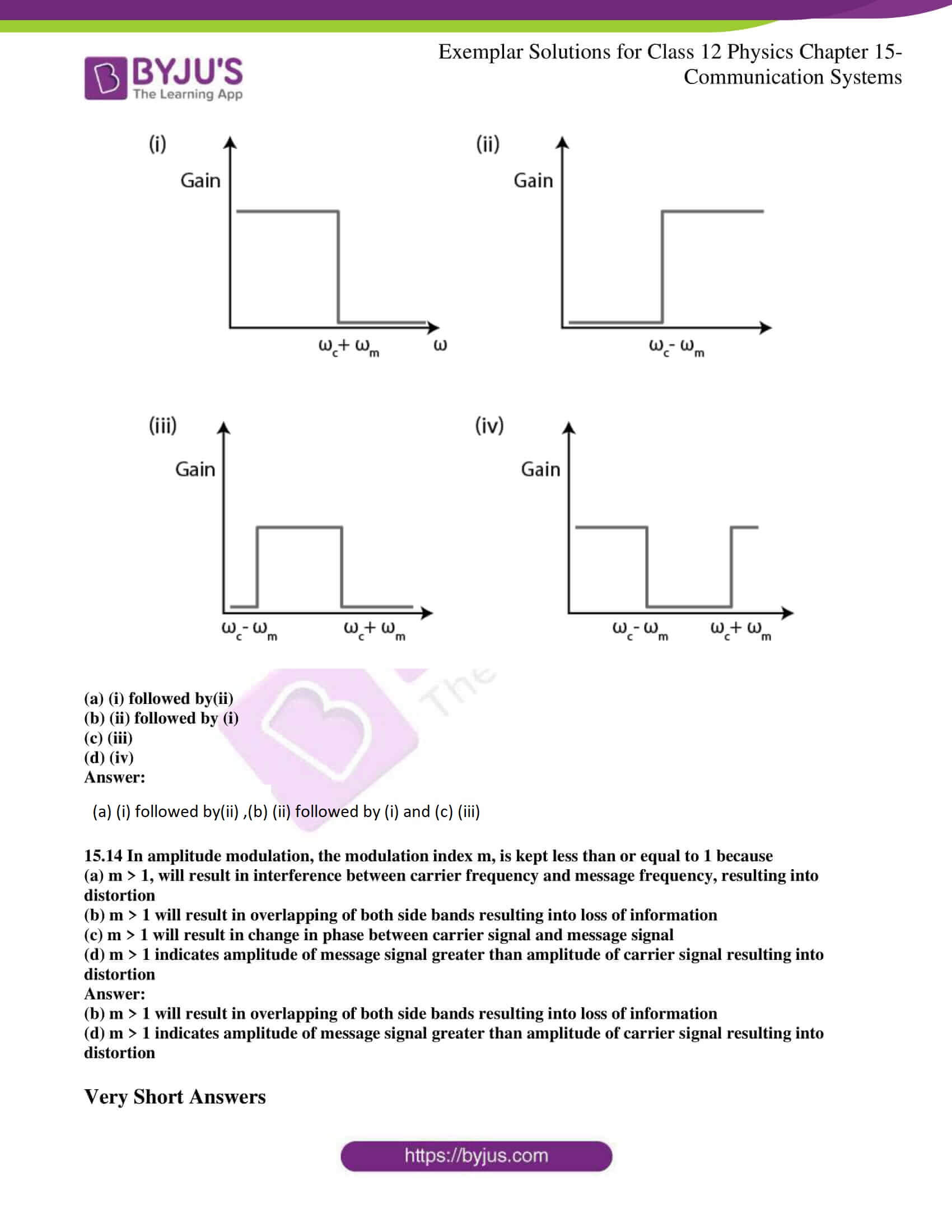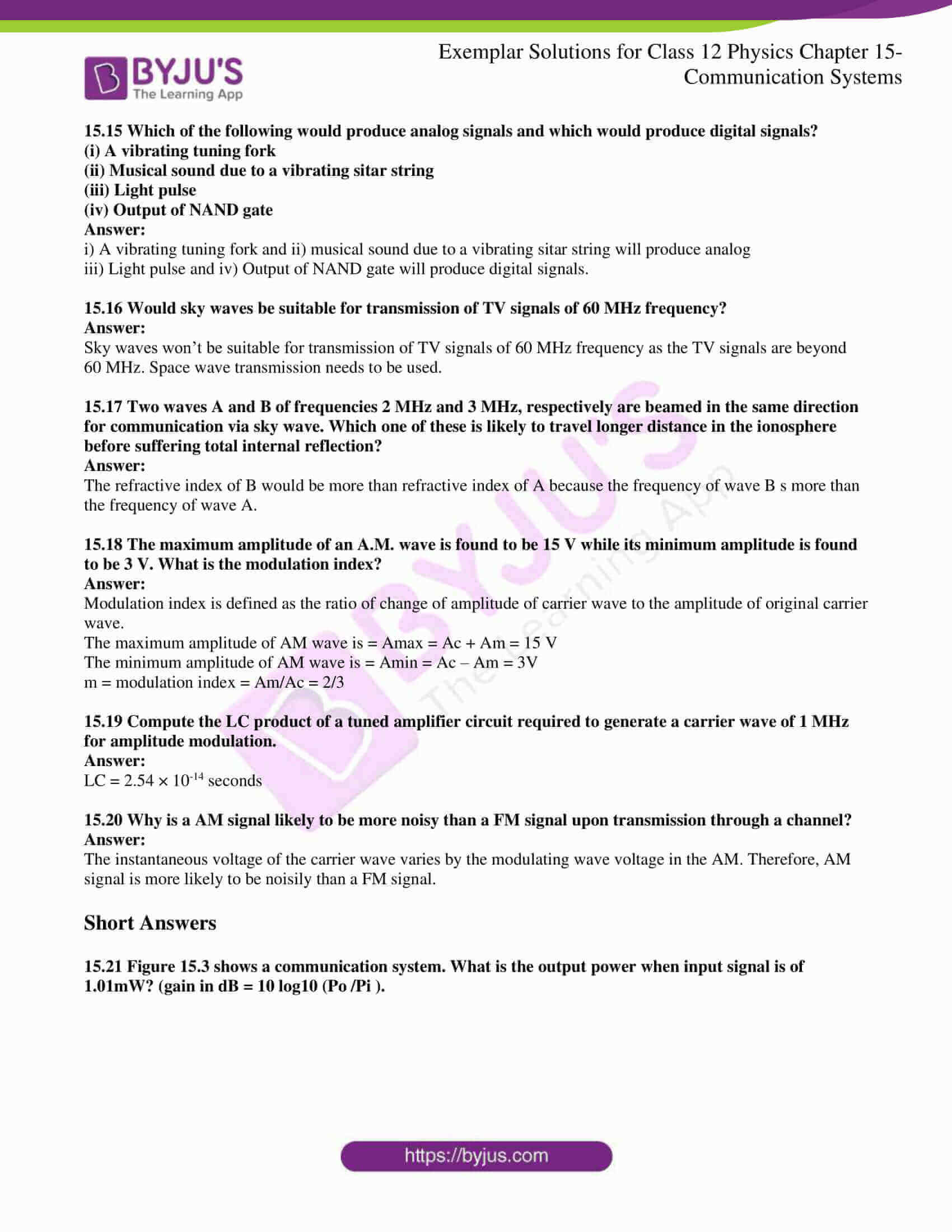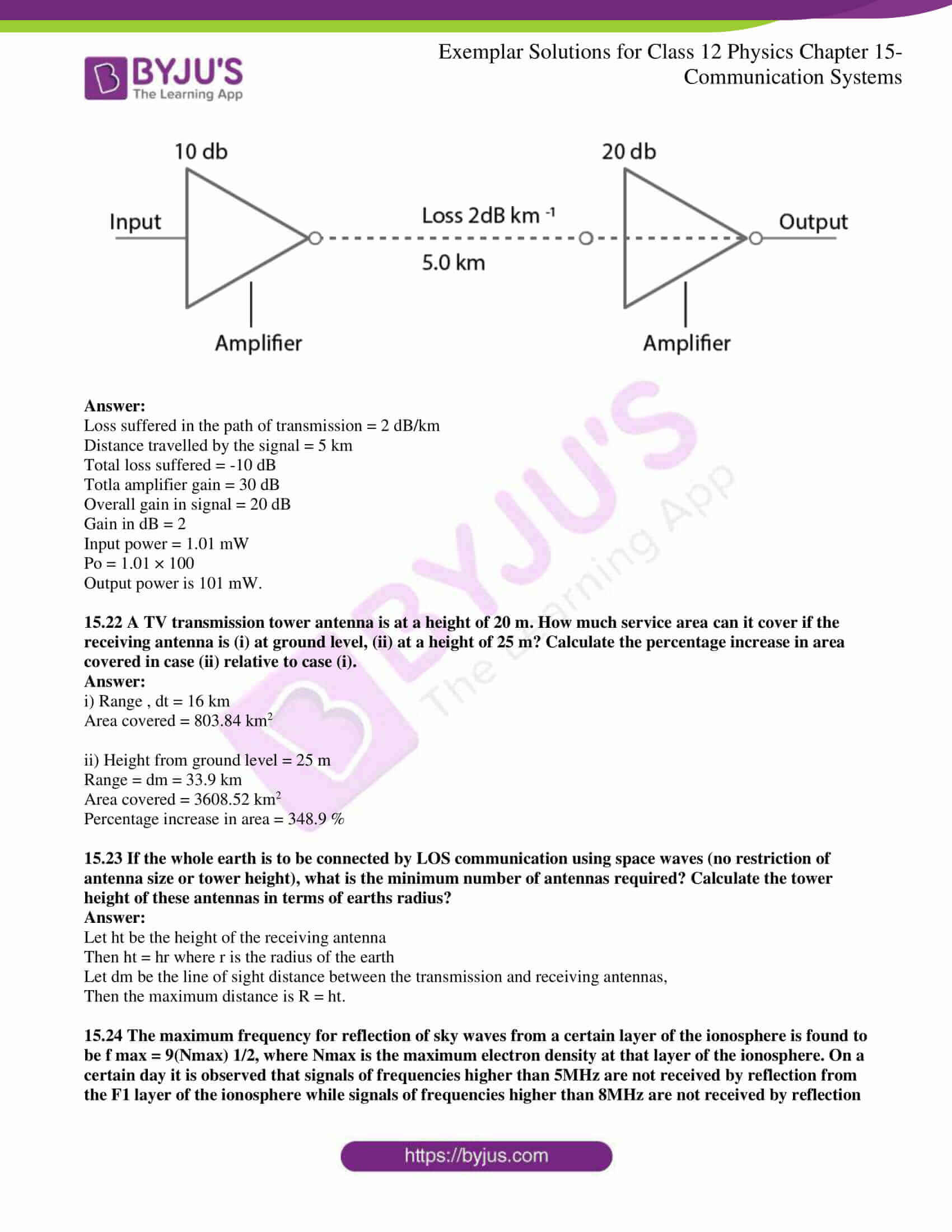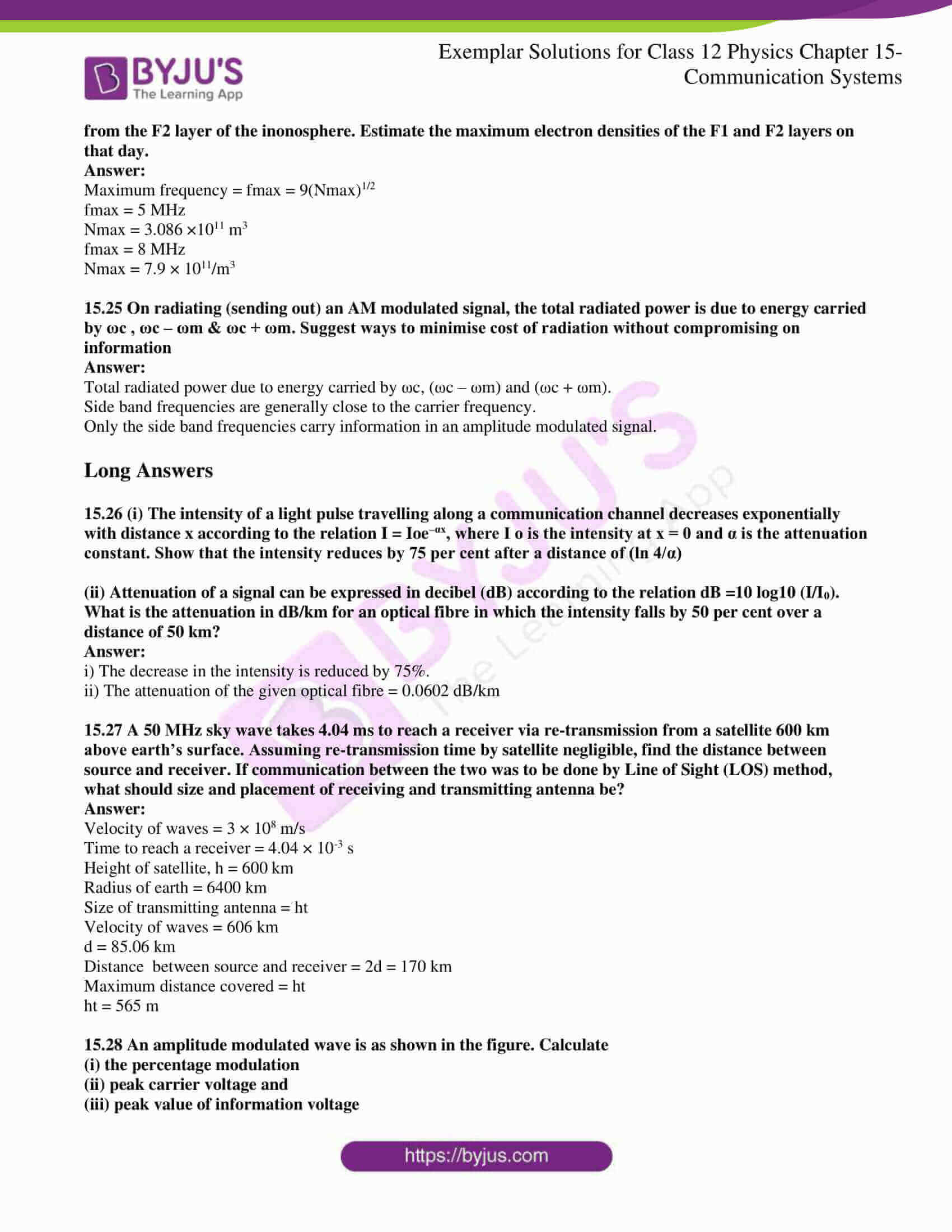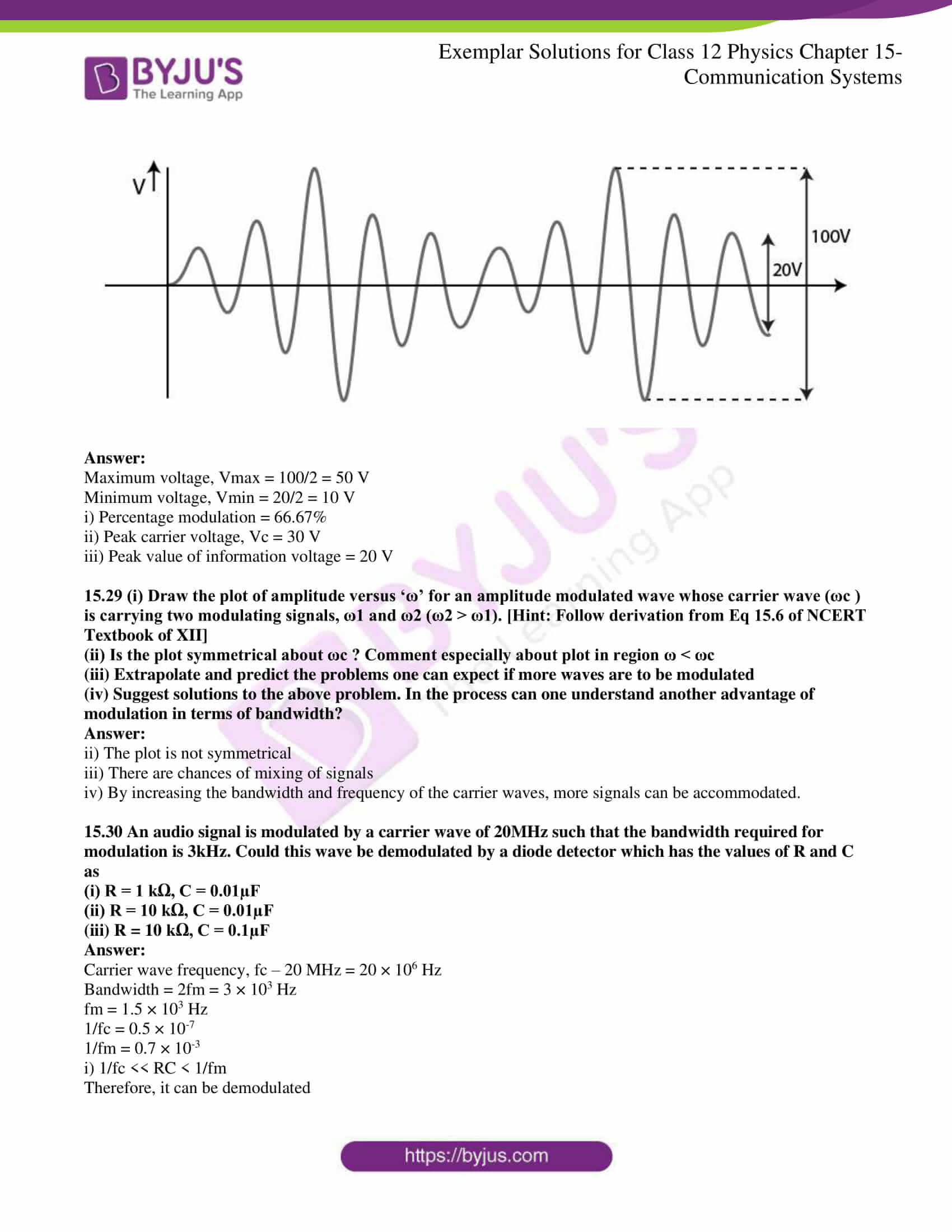### Multiple Choice Questions I

15.1 Three waves A, B and C of frequencies 1600 kHz, 5 MHz and 60 MHz, respectively are to be transmitted from one place to another. Which of the following is the most appropriate mode of communication:

(a) A is transmitted via space wave while B and C are transmitted via skywave

(b) A is transmitted via ground wave, B via skywave and C via space wave

(c) B and C are transmitted via ground wave while A is transmitted via skywave

(d) B is transmitted via ground wave while A and C are transmitted via space wave

(b) A is transmitted via ground wave, B via skywave and C via space wave

15.2 A 100m long antenna is mounted on a 500m tall building. The complex can become a transmission tower for waves with λ

(a) ~ 400 m

(b) ~ 25 m

(c) ~ 150 m

(d) ~ 2400 m

(a) ~ 400 m

15.3 A 1 KW signal is transmitted using a communication channel which provides attenuation at the rate of – 2dB per km. If the communication channel has a total length of 5 km, the power of the signal received is [gain in dB = 10 log P0/P1]

(a) 900 W

(b) 100 W

(c) 990 W

(d) 1010 W

(b) 100 W

15.4 A speech signal of 3 kHz is used to modulate a carrier signal of frequency 1 MHz, using amplitude modulation. The frequencies of the sidebands will be

(a) 1.003 MHz and 0.997 MHz

(b) 3001 kHz and 2997 kHz

(c) 1003 kHz and 1000 kHz

(d) 1 MHz and 0.997 MHz

(a) 1.003 MHz and 0.997 MHz

15.5 A message signal of frequency ωm is superposed on a carrier wave of frequency ωc to get an amplitude modulated wave (AM). The frequency of the AM wave will be

(a) ωm

(b) ωc

(c) ωc + ωm/2

(d) ωc – ωm/2

(b) ωc

15.6 I-V characteristics of four devices are shown in the figure.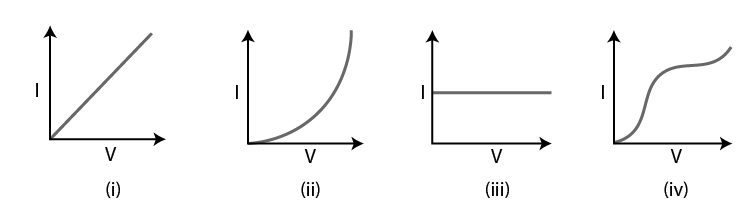Identify devices that can be used for modulation:

(a) ‘i’ and ‘iii’

(b) only ‘iii’

(c) ‘ii’ and some regions of ‘iv’

(d) All the devices can be used.

(c) ‘ii’ and some regions of ‘iv’

15.7 A male voice after modulation-transmission sounds like that of a female to the receiver. The problem is due to

(a) poor selection of modulation index (selected 0 < m < 1)

(b) poor bandwidth selection of amplifiers

(c) poor selection of carrier frequency

(d) loss of energy in transmission

(b) poor bandwidth selection of amplifiers

15.8 A basic communication system consists of

(A) transmitter

(B) information source

(C) user of information

(D) channel

Choose the correct sequence in which these are arranged in a basic communication system:

(a) ABCDE

(c) BDACE

15.9 Identify the mathematical expression for amplitude modulated wave:

(a) Ac sin [{ωc + k1vm(t)}t + φ ]

(b) Ac sin {ωc t + φ + k2 vm(t)}

(c) {Ac + k2 vm(t)} sin (ωc t + φ )

(d) Ac vm(t) sin (ωc t + φ )

(c) {Ac + k2 vm(t)} sin (ωc t + φ )

### Multiple Choice Questions II

15.10 An audio signal of 15kHz frequency cannot be transmitted over long distances without modulation because

(a) the size of the required antenna would be at least 5 km which is not convenient

(b) the audio signal can not be transmitted through sky waves

(c) the size of the required antenna would be at least 20 km, which is not convenient

(d) effective power transmitted would be very low, if the size of the antenna is less than 5 km

(a) the size of the required antenna would be at least 5 km which is not convenient

(b) the audio signal can not be transmitted through sky waves

(d) effective power transmitted would be very low, if the size of the antenna is less than 5 km

15.11 Audio sine waves of 3 kHz frequency are used to amplitude modulate a carrier signal of 1.5 MHz. Which of the following statements are true?

(a) The sideband frequencies are 1506 kHz and 1494 kHz

(b) The bandwidth required for amplitude modulation is 6kHz

(c) The bandwidth required for amplitude modulation is 3 MHz

(d) The sideband frequencies are 1503 kHz and 1497 kHz.

(b) The bandwidth required for amplitude modulation is 6kHz

(d) The sideband frequencies are 1503 kHz and 1497 kHz.

15.12 A TV transmission tower has a height of 240 m. Signals broadcast from this tower will be received by LOS communication at a distance of (assume the radius of the earth to be 6.4 × 106 m)

(a) 100 km

(b) 24 km

(c) 55 km
(d) 50 km

(b) 24 km

(c) 55 km
(d) 50 km

15.13 The frequency response curve in the figure for the filter circuit used for production of AM wave should be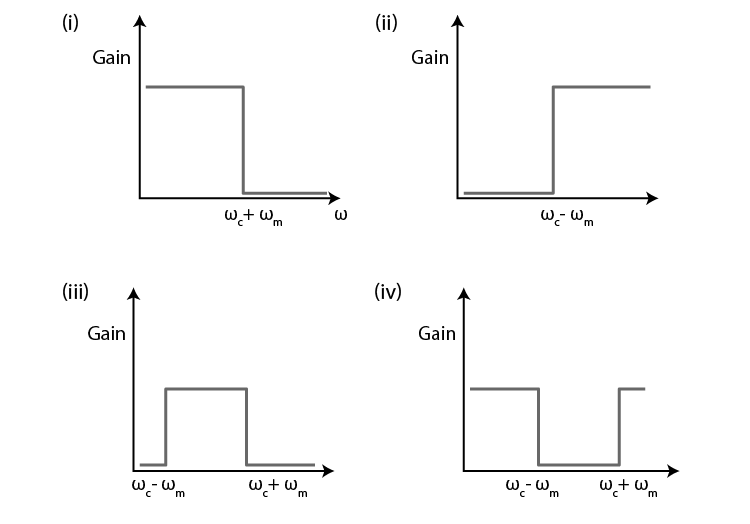(a) (i) followed by(ii)

(b) (ii) followed by (i)

(c) (iii)

(d) (iv)

(a) (i) followed by(ii)

(b) (ii) followed by (i)

(c) (iii)

15.14 In amplitude modulation, the modulation index m, is kept less than or equal to 1 because

(a) m > 1, will result in interference between the carrier frequency and message frequency, resulting in distortion

(b) m > 1 will result in overlapping of both sidebands resulting into loss of information

(c) m > 1 will result in a change in phase between the carrier signal and the message signal

(d) m > 1 indicates the amplitude of message signal greater than the amplitude of the carrier signal resulting in distortion

(b) m > 1 will result in overlapping of both sidebands resulting into loss of information

(d) m > 1 indicates the amplitude of message signal greater than the amplitude of the carrier signal resulting in distortion

15.15 Which of the following would produce analogue signals and which would produce digital signals?

(i) A vibrating tuning fork

(ii) Musical sound due to a vibrating sitar string

(iii) Light pulse

(iv) Output of NAND gate

i) A vibrating tuning fork and ii) musical sound due to a vibrating sitar string will produce analogue

iii) Light pulse and iv) Output of NAND gate will produce digital signals.

15.16 Would sky waves be suitable for transmission of TV signals of 60 MHz frequency?

Skywaves won’t be suitable for transmission of TV signals of 60 MHz frequency as the TV signals are beyond 60 MHz. Space wave transmission needs to be used.

15.17 Two waves A and B of frequencies 2 MHz and 3 MHz, respectively are beamed in the same direction for communication via skywave. Which one of these is likely to travel a longer distance in the ionosphere before suffering total internal reflection?

The refractive index of B would be more than a refractive index of A because the frequency of wave B s more than the frequency of wave A.

15.18 The maximum amplitude of an A.M. wave is found to be 15 V while its minimum amplitude is found to be 3 V. What is the modulation index?

Modulation index is defined as the ratio of change of amplitude of carrier wave to the amplitude of the original carrier wave.

The maximum amplitude of AM wave is = Amax = Ac + Am = 15 V

The minimum amplitude of the AM wave is = Amin = Ac – Am = 3V

m = modulation index = Am/Ac = 2/3

15.19 Compute the LC product of a tuned amplifier circuit required to generate a carrier wave of 1 MHz for amplitude modulation.

LC = 2.54 × 10-14 seconds

15.20 Why is an AM signal likely to be noisier than an FM signal upon transmission through a channel?

The instantaneous voltage of the carrier wave varies by the modulating wave voltage in the AM. Therefore, the AM signal is more likely to be noisily than an FM signal.

15.21 Figure 15.3 shows a communication system. What is the output power when the input signal is of 1.01mW? (gain in dB = 10 log10 (Po /Pi ).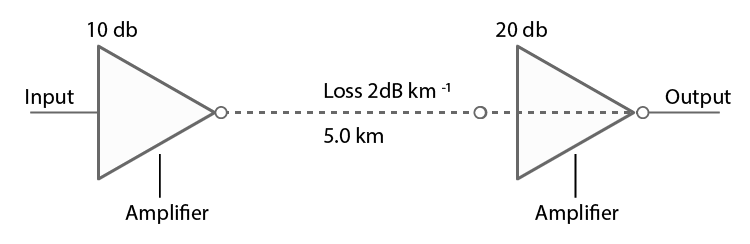Loss suffered in the path of transmission = 2 dB/km

Distance travelled by the signal = 5 km

Total loss suffered = -10 dB

Totla amplifier gain = 30 dB

Overall gain in signal = 20 dB

Gain in dB = 2

Input power = 1.01 mW

Po = 1.01 × 100

Output power is 101 mW.

15.22 A TV transmission tower antenna is at a height of 20 m. How much service area can it cover if the receiving antenna is (i) at ground level, (ii) at a height of 25 m? Calculate the percentage increase in area covered in case (ii) relative to case (i).

i) Range , it = 16 km

Area covered = 803.84 km2

ii) Height from ground level = 25 m

Range = dm = 33.9 km

Area covered = 3608.52 km2

Percentage increase in area = 348.9 %

15.23 If the whole earth is to be connected by LOS communication using space waves (no restriction of antenna size or tower height), what is the minimum number of antennas required? Calculate the tower height of these antennas in terms of earths radius?

Let ht be the height of the receiving antenna

Then ht = hr where r is the radius of the earth

Let dm be the line of sight distance between the transmission and receiving antennas,

Then the maximum distance is R = ht.

15.24 The maximum frequency for reflection of sky waves from a certain layer of the ionosphere is found to be f max = 9(Nmax) 1/2, where Nmax is the maximum electron density at that layer of the ionosphere. On a certain day, it is observed that signals of frequencies higher than 5MHz are not received by reflection from the F1 layer of the ionosphere while signals of frequencies higher than 8MHz are not received by reflection from the F2 layer of the ionosphere. Estimate the maximum electron densities of the F1 and F2 layers on that day.

Maximum frequency = fmax = 9(Nmax)1/2

fmax = 5 MHz

Nmax = 3.086 ×1011 m3

fmax = 8 MHz

Nmax = 7.9 × 1011/m3

15.25 On radiating (sending out) an AM modulated signal, the total radiated power is due to energy carried by ωc, ωc – ωm & ωc + ωm. Suggest ways to minimise the cost of radiation without compromising on information

Total radiated power due to energy carried by ωc, (ωc – ωm) and (ωc + ωm).

Sideband frequencies are generally close to the carrier frequency.

Only the sideband frequencies carry information in an amplitude modulated signal.

15.26 (i) The intensity of a light pulse travelling along a communication channel decreases exponentially with distance x according to the relation I = Ioe–αx, where I o is the intensity at x = 0 and α is the attenuation constant. Show that the intensity reduces by 75 per cent after a distance of (ln 4/α)

(ii) Attenuation of a signal can be expressed in decibel (dB) according to the relation dB =10 log10 (I/I0). What is the attenuation in dB/km for an optical fibre in which the intensity falls by 50 per cent over a distance of 50 km?

i) The decrease in the intensity is reduced by 75%.

ii) The attenuation of the given optical fibre = 0.0602 dB/km

15.27 A 50 MHz sky wave takes 4.04 ms to reach a receiver via re-transmission from a satellite 600 km above earth’s surface. Assuming re-transmission time by satellite negligible, find the distance between source and receiver. If communication between the two was to be done by Line of Sight (LOS) method, what should size and placement of receiving and transmitting antenna be?

Velocity of waves = 3 × 108 m/s

Time to reach a receiver = 4.04 × 10-3 s

Height of satellite, h = 600 km

Radius of earth = 6400 km

Size of transmitting antenna = ht

Velocity of waves = 606 km

d = 85.06 km

Distance between source and receiver = 2d = 170 km

Maximum distance covered = ht

ht = 565 m

15.28 An amplitude modulated wave is as shown in the figure. Calculate

(i) the percentage modulation

(ii) peak carrier voltage and

(iii) peak value of information voltage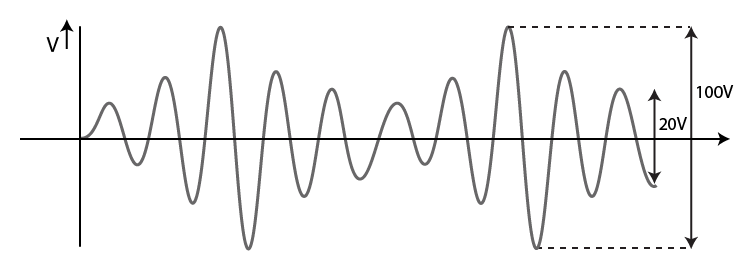Maximum voltage, Vmax = 100/2 = 50 V

Minimum voltage, Vmin = 20/2 = 10 V

i) Percentage modulation = 66.67%

ii) Peak carrier voltage, Vc = 30 V

iii) Peak value of information voltage = 20 V

15.29 (i) Draw the plot of amplitude versus ‘ω’ for an amplitude modulated wave whose carrier wave (ωc ) is carrying two modulating signals, ω1 and ω2 (ω2 > ω1). [Hint: Follow derivation from Eq 15.6 of NCERT Textbook of XII]

(ii) Is the plot symmetrical about ωc? Comment especially about plot in region ω < ωc

(iii) Extrapolate and predict the problems one can expect if more waves are to be modulated

(iv) Suggest solutions to the above problem. In the process can one understand another advantage of modulation in terms of bandwidth?

ii) The plot is not symmetrical

iii) There are chances of mixing of signals

iv) By increasing the bandwidth and frequency of the carrier waves, more signals can be accommodated.

15.30 An audio signal is modulated by a carrier wave of 20MHz such that the bandwidth required for modulation is 3kHz. Could this wave be demodulated by a diode detector which has the values of R and C as

(i) R = 1 kΩ, C = 0.01µF

(ii) R = 10 kΩ, C = 0.01µF

(iii) R = 10 kΩ, C = 0.1µF

Carrier wave frequency, fc – 20 MHz = 20 × 106 Hz

Bandwidth = 2fm = 3 × 103 Hz

fm = 1.5 × 103 Hz

1/fc = 0.5 × 10-7

1/fm = 0.7 × 10-3

i) 1/fc << RC < 1/fm

Therefore, it can be demodulated

ii) 1/fc << RC << 1/fm

Therefore, it can be demodulated

iii) 1/fc < RC

Therefore, it cannot be demodulated

Communication systems are a collection of systems used for transmission, connection, communication and interconnection. These systems are categorically arranged into three different types based on uses, such as media, technology and application area. Sensors, transducers, emitters and amplifiers are all examples of modern technology which are used as components in the majority of modern devices.

1. E-mail
2. Internet
3. Television
5. Computer

### Concepts Discussed in Chapter 15 Communication System

1. Introduction
2. Elements of a Communication System
3. Basic Terminology Used in Electronic Communication Systems
4. Bandwidth of Signals
5. Bandwidth of Transmission Medium
6. Propagation of Electromagnetic Waves
1. Ground wave
2. Skywaves
3. Space wave
7. Modulation and Its Necessity
8. Size of the Antenna or Aerial
9. Effective Power Radiated by an Antenna Ex 15.7.3 – Mixing up of Signals from Different Transmitters
10. Amplitude Modulation
11. Production of Amplitude Modulated Wave
12. Detection of Amplitude Modulated Wave

BYJU’S is reforming the education sector of the country through its innovative methodology that uses videos, animations and infographics to teach the students. Our interactive model of teaching helps them learn more effectively than traditional teaching methods.